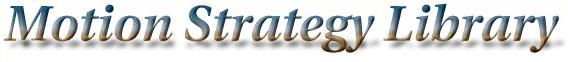Main Page   Namespace List   Class Hierarchy   Compound List   File List   Compound Members   File Members

# MSLVertex Class Reference

`#include <graph.h>`

List of all members.

## Public Methods

MSLVector State () const
The state to which this node corresponds. More...

list< MSLEdge * > Edges () const
Return all of the incident edges. More...

double Cost () const
A cost value, useful in some algorithms. More...

void SetCost (const double &x)
A cost value, useful in some algorithms. More...

void SetID (const int &i)
Change the vertex ID. More...

int ID () const
Get the vertex ID. More...

void Mark ()
A cost value, useful in some algorithms. More...

void Unmark ()
A cost value, useful in some algorithms. More...

bool IsMarked () const
A cost value, useful in some algorithms. More...

MSLVertex ()
MSLVertex (const MSLVector &x)
~MSLVertex ()

## Friends

class MSLEdge
class MSLGraph
istream & operator>> (istream &is, MSLVertex &n)
ostream & operator<< (ostream &os, const MSLVertex &n)

## Constructor & Destructor Documentation

 MSLVertex::MSLVertex ( )

 MSLVertex::MSLVertex ( const MSLVector & x )

 MSLVertex::~MSLVertex ( )

## Member Function Documentation

 double MSLVertex::Cost ( ) const` [inline]`
 A cost value, useful in some algorithms.

 list MSLVertex::Edges ( ) const` [inline]`
 Return all of the incident edges.

 int MSLVertex::ID ( ) const` [inline]`
 Get the vertex ID.

 bool MSLVertex::IsMarked ( ) const` [inline]`
 A cost value, useful in some algorithms.

 void MSLVertex::Mark ( ) ` [inline]`
 A cost value, useful in some algorithms.

 void MSLVertex::SetCost ( const double & x ) ` [inline]`
 A cost value, useful in some algorithms.

 void MSLVertex::SetID ( const int & i ) ` [inline]`
 Change the vertex ID.

 MSLVector MSLVertex::State ( ) const` [inline]`
 The state to which this node corresponds.

 void MSLVertex::Unmark ( ) ` [inline]`
 A cost value, useful in some algorithms.

## Friends And Related Function Documentation

 friend class MSLEdge` [friend]`

 friend class MSLGraph` [friend]`

 ostream& operator<< ( ostream & os, const MSLVertex & n ) ` [friend]`

 istream& operator>> ( istream & is, MSLVertex & n ) ` [friend]`

The documentation for this class was generated from the following files: Motion Strategy Library

Web page maintained by Steve LaValle